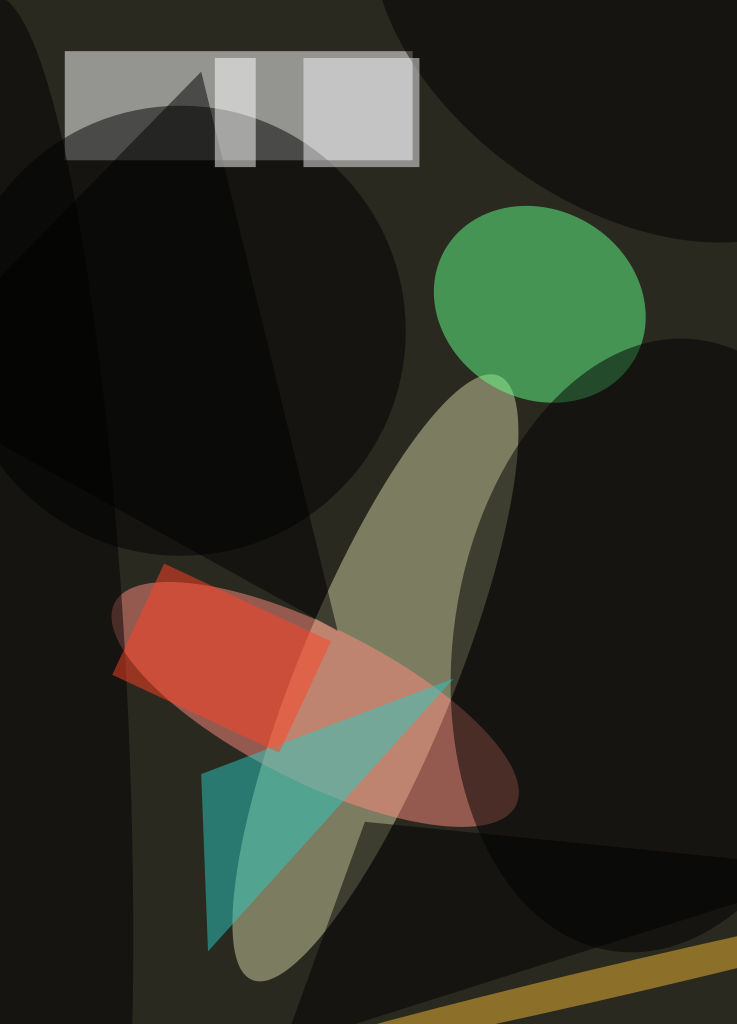A proposed method of measuring gravitational acceleration would involve the application of polarization spectroscopy to an ultracold, vertically moving cloud of atoms (an atomic fountain). A related proposed method involving measurements of absorption of light pulses like those used in conventional atomic interferometry would yield an estimate of the number of atoms participating in the interferometric interaction.

The basis of the first-mentioned proposed method is that the rotation of polarization of light is affected by the acceleration of atoms along the path of propagation of the light. The rotation of polarization is associated with a phase shift: When an atom moving in a laboratory reference interacts with an electromagnetic wave, the energy levels of the atom are Doppler-shifted, relative to where they would be if the atom were stationary. The Doppler shift gives rise to changes in the detuning of the light from the corresponding atomic transitions. This detuning, in turn, causes the electromagnetic wave to undergo a phase shift that can be measured by conventional means. One would infer the gravitational acceleration and/or the gradient of the gravitational acceleration from the phase measurements.

The figure depicts the optical layout of a version of an apparatus that would be used in the proposed method to measure the gravitational acceleration. (A slightly different version would be used to measure a gradient in the gravitational acceleration.) Also shown is a diagram of the relevant energy levels of atoms of an element suitable for use in these methods: The element must be one that has a L-shaped energy-level scheme as depicted here. A linearly polarized laser beam would impinge on a first polarizing beam splitter (PBS1), which would divide the beam into two beams of equal power. By use of quarter-wave plates, the two beams would be given opposite circular polarizations. One of the two beams would be steered to propagate upward, the other to propagate downward along a vertical tube containing an ultracold cloud of the atoms in question.

After propagating through the cloud, the beams would be converted back to linear polarizations by the same quarter-wave plates, then recombined in PBS1 to produce an output beam, which would be steered through a quarter-wave plate oriented at 45° with respect to the original linear polarization. This quarter-wave plate would transform the beam into a linearly polarized beam, the polarization direction of which would differ from that of the original laser light by an angle, f, that would depend on the velocity of the atoms. A second polarizing beam splitter (PBS2), also oriented at 45° with respect to the original polarization, would split the beam into two parts that would go to two photodiodes (d1 and d2). The output currents of the two photodiodes would be proportional to

(1/2)[P++ P- ± 2(P+P-)1/2sin(2Φ)],

where P+ and P- denote the powers of the two opposite circularly polarized beams. The sum of the two photocurrents would be proportional to the total output power, while the difference between them would give information on the polarization-rotation angle,Φ.

A lengthy mathematical derivation that must be omitted here for the sake of brevity leads to an equation for dependence of f on a number of variables that include time (t) and the gravitational acceleration (g). The equation could be used to infer g from the measurements. A further derivation shows that the signal-to-noise ratio would be maximized by filtering the photodetector outputs via an appropriate time-dependent function and by choosing an optimum number of photons, which turns out to equal the number of atoms interacting with the light.

In the second-mentioned proposed method (the one for estimating the number of atoms participating in interferometric interactions), one would exploit the connection between the number of photons and the atomic state-population in a Raman configuration. As in the method described above, the atoms to be probed must have a L-shaped energy-level scheme. One would utilize light pulses that are already used to prepare the atomic cloud in a particular quantum state in conventional atomic interferometer schemes. Yet another mathematical derivation shows that the difference between the numbers of photons of two counter-propagating electromagnetic fields used to generate the pulses is related to the number of participating atoms. This method of atomic-population measurement may offer sensitivity greater than is offered by any prior method. This method would increase the sensitivities afforded by pre-existing atomic-interferometry schemes, without need to change those schemes significantly. Moreover, the measurements of the light pulses may also yield rough estimates of the expected interferometric signals.

This work was done by Andrey Matsko, Nan Yu, and Lute Maleki of Caltech for NASA's Jet Propulsion Laboratory.

NPO-30715##### Photonics Tech Briefs Magazine

This article first appeared in the January, 2004 issue of Photonics Tech Briefs Magazine.

Read more articles from the archives here.